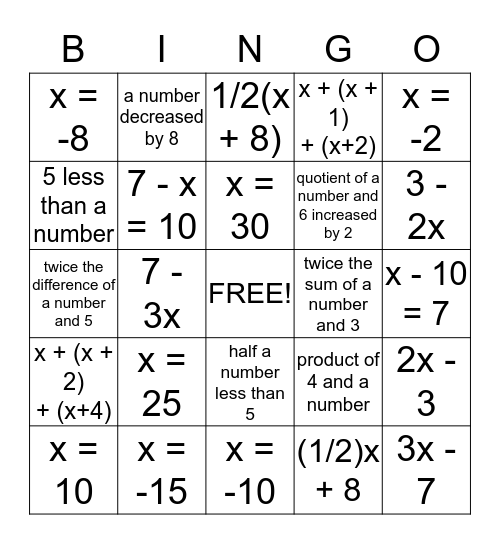# Algebraic Expressions ReviewThis bingo card has a free space and 24 words: x = 25, x = -15, x = 30, x = 10, x = -2, x = -8, 5 less than a number, a number decreased by 8, twice the sum of a number and 3, half a number less than 5, quotient of a number and 6 increased by 2, twice the difference of a number and 5, product of 4 and a number, 2x - 3, 7 - x = 10, (1/2)x + 8, x - 10 = 7, 1/2(x + 8), 3 - 2x, 7 - 3x, 3x - 7, x + (x + 2) + (x+4), x + (x + 1) + (x+2) and x = -10.

## Play Online